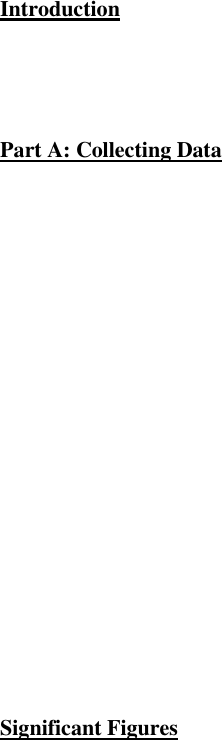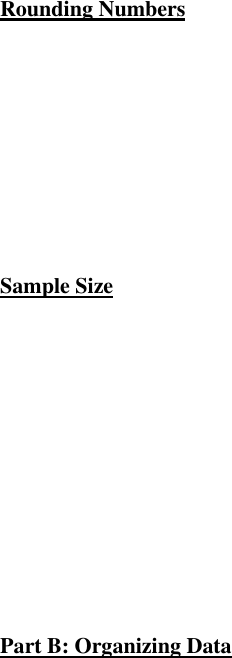# BIO120H1 Chapter Notes - Chapter B: Significant Figures, Scale Factor, Scientific Notation

277 views7 pages
School
Department
CourseBIO120: Adaptation and Biodiversity Lab Manual (Appendix B
Introduction to Statistics)
In association with Test 2
- After reading this, you should be able to:
o Appreciate why and how biologists use statistics
o Understand the use of scientific notation and significant figure
o Distinguish between the kinds of measurement data
o Understand how descriptive statistics mean, median, mode, range, variance, standard
deviation, and standard error are used
o Describe the relationship between hypothesis testing and statistics
o Choose which test of significance is appropriate for what kind of data
o Perform and interpret a t test and x2 test
Introduction
- Statistics give special meaning to significance, error, hypothesis, and variance can lead to
confusion for many students unless special attention is paid to distinct meaning of statistical
words
Part A: Collecting Data
- Data are information obtained by measurements and from which conclusions can be drawn
- Measurement plays major role in scientific method
- Measurement Error
o If a single object is measured repeatedly, values obtained aren’t all identical; some
variation exists in repeated measurements
o Measurement error reflects discrepancy between our repeated measurements and true
value of object being measured
o Potential sources of air must be eliminated when designing and conducting experiments,
or to compensate for them in data analysis
- Precision and Accuracy
o Measurements have associated with them both precision and accuracy
o Precision is repeatability of the measurement; if single measurement when repeated
shows little variation, it has high precision; if it shows great variation, it has low
precision
o Measurement can be precise but not be close to true value of object being measured
precise but source of error exists that caused measurements to differ consistently from
true value
o Accuracy is tendency for measured value to be close to, or approximate, the true accepted
value
Significant Figures
- Single measurement consists of three parts:
o Unit measured
o Scale factor showing relative magnitude of value
o Significant figures
Unlock document

This preview shows pages 1-2 of the document.
Unlock all 7 pages and 3 million more documents.- Number of significant figures depends upon accuracy of measuring instrument
- As a general rule, number of significant figures should reflect numbers known to be correct, and
no figures which are known to be correct should be omitted
- To avoid confusion between scale factors and significant figures, measurements can be expressed
using scientific notation
- When performing arithmetic operations, assume following when estimating significance of
results:
o When multiplying and dividing numbers, results should have no more reliability than
initial value which has least number of significant figures
o When adding and subtracting numbers, result should contain only as many decimal
places as did the initial value which as least number of decimal places
Rounding Numbers
- Do not change digit to be rounded if it is followed by a digit less than five
- If the digit to be rounded is followed by a digit greater than five, followed by digits greater than
zero, increase it by one
- If digit to be rounded is followed by a five followed by zeros, then
o If digit is even, leave it unchanged
o If digit is odd, increase it by one
o This is done so that one half of the time the figure will be rounded higher and the other
half will be rounded lower, avoiding error in the procedure
Sample Size
- A sample is a subset of a population and consists of only a small proportion of all the individuals
in the population
- To adequately describe population, randomly selected sampling units are examined and hope that
units represent range and frequency of values present in population
- Information about population is statistically inferred from information in our sample
- In general, larger samples give more reliable information about population
- Size of sample must always be reported allows scientist to judge importance of reported
findings for themselves
- Sample size is typically abbreviated with a small “n”; size of population is denoted as “N”
- You can’t increase sample size by endlessly repeating measurements on same sampling unit
must measure more units to increase sample size
Part B: Organizing Data
- All data have certain characteristics or attributes; before data can be analyzed, they’re grouped
according to qualitative or quantitative nature
- Qualitative attributes (i.e. sex, hair colour, presence of a particular disease) are easy to group as
only a definite number of possibilities exist
- Quantitative data may be of two types:
o Discrete
Those in which only whole units can be counted or measured
o Continuous
Those in which measurement can be a fractional part depending on the fineness
of measurement
- There are two common methods of presenting quantitative data
Unlock document

This preview shows pages 1-2 of the document.
Unlock all 7 pages and 3 million more documents.

# Get access

\$10 USD/m
Billed \$120 USD annually
Homework Help
Class Notes
Textbook Notes